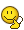# Calculating the Total Energy of a lattice w/ the Madelung constant

• B
etotheipi
For an ionic lattice, the contribution to the electric potential energy from a single ion will be ##U_i = \sum_{j\neq i} U_{ij}##, which can be expressed as\begin{align*}U_i &= -6 \left( \frac{z_+ z_- e^2}{4\pi \varepsilon_0 r_0} \right) + 12 \left( \frac{z_+ z_- e^2}{4\pi \varepsilon_0 \sqrt{2} r_0} \right) - 8 \left( \frac{z_+ z_- e^2}{4\pi \varepsilon_0 \sqrt{3} r_0} \right) + \dots \\ \\ &= -\frac{z_+ z_- e^2}{4\pi \varepsilon_0 r_0} \left(6 - \frac{12}{\sqrt{2}} + \frac{8}{\sqrt{3}} + \dots \right) := -\frac{z_+ z_- e^2}{4\pi \varepsilon_0 r_0} M \end{align*}where ##M## is the Madelung constant, ##r_0## the ionic spacing, and ##q_+ = z_+ e##, ##q_- = -z_- e##. The next line in the book ("why chemical reactions happen", Keeler/Wothers - two very distinguished chemists!) states that the total energy, per mole, of the lattice can be obtained with $$U = \sum_i U_i = -\frac{z_+ z_- e^2}{4\pi \varepsilon_0 r_0} M N_A$$However, I don't think this is right. Specifically, it seems like they are double counting interactions, when in fact we should only be counting one out of ##U_{ij}## and ##U_{ji}## each time. I would have thought the total energy be half of this$$U = \frac{1}{2} \sum_i \sum_{j \neq i} U_{ij} = -\frac{z_+ z_- e^2}{8\pi \varepsilon_0 r_0} M N_A$$The thing is, this factor of a half is absent from other references too (like here).

So I wondered if anyone else would agree, or if I'm missing something (N.B. for now, I'm ignoring the repulsive ##\frac{B}{r^n}## contribution to the energy). Thanks!

## Answers and Replies

etotheipi
Oh hang on, I suspect they're talking about ##N_A## of ##M^+## and ##N_A## of ##A^-## (i.e. one mole of ##MA##), whilst I'm talking about ##N_A/2## of ##M^+## and ##N_A/2## of ##A^-## (i.e. one mole of total ions). There's the implicit factor of two. Whoops